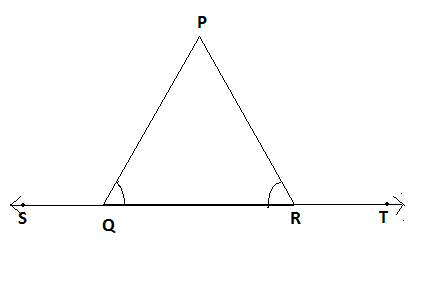QUESTION

# In the figure, $\angle PQR = \angle PRQ$ , then prove that $\angle PQS = \angle PRT$.Hint: In this question, we use the concept of properties of triangle. We use the property that an exterior angle of a triangle is equal to the sum of the opposite interior angles.

Complete Step-by-Step solution:
Given that $\angle PQR = \angle PRQ$
We have to prove that $\angle PQS = \angle PRT$
Now, we can see $\angle PQS$ and $\angle PRT$ are exterior angles. So, we use the basic property of a triangle that is that the exterior angle of a triangle is equal to the sum of the opposite interior angles.
We can see $\angle PRT$ is exterior angle and $\angle PQR,\angle QPR$ are opposite interior angles.
So, $\angle PQR + \angle QPR = \angle PRT..............\left( 1 \right)$
Now, we can see $\angle PQS$ is exterior angle and $\angle PRQ,\angle QPR$ are opposite interior angles.
So, $\angle PRQ + \angle QPR = \angle PQS..............\left( 2 \right)$
From (1) and (2) equation eliminate $\angle QPR$
$\Rightarrow \angle PRT - \angle PQR = \angle PQS - \angle PRQ.............\left( 3 \right)$
Now, given in question $\angle PQR = \angle PRQ$
From (3) equation,
$\Rightarrow \angle PRT = \angle PQS$
Hence proved, $\angle PQS = \angle PRT$.

Note: We can solve the above question by two different ways. First way we already mention in above and in second way, we have to use the property of linear pairs. We know pairs of adjacent angles whose measures add up to form a straight angle is known as a linear pair.
$\Rightarrow \angle PQS + \angle PQR = {180^0}$ (Linear pair)………….. (1)
$\Rightarrow \angle PRT + \angle PRQ = {180^0}$ (Linear pair)………….. (2)
After solving both equations we will get $\angle PQS = \angle PRT$